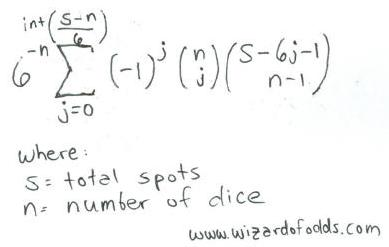# 骰宝附录

## 简介• 低:

如果不算triple三颗同点的状况, 这个押注没有赌场优势。三颗同点在1点, 2点, 或3点时的机率为3/216. 总点数在3点到10点之间的机率为1/2, 或 108/216. 所以赢注的机率为108/216 - 3/216 = 105/216. 因此玩家的优势 为(105/216)*(+1) + (111/216)*(-1) = -6/216 =~-2.78%.

• ⾼: 参⻅Low低

• 特定点数:

摇出0颗骰⼦特定点数的机率为 (5/6)^3 =125/216.
摇出1颗骰⼦特定点数的机率为 3*(1/6)^1*(5/6)^2 = 75/216.
摇出2颗骰⼦特定点数的机率为 3*(1/6)^2*(5/6)^1 = 15/216.
摇出3颗骰⼦特定点数的机率为 (1/6)^3 = 1/216.因此玩家的优势为(125/216)*(-1) + (75/216)*(+1)+ (15/216)*(+2) + (1/216)*(+3) = - 17/216 =~-7.780%.

• 总点数4点:

共有3种形式摇出4点: (1+1+2, 1+2+1, 2+1+1). 因此玩家的优势为 3/216*(+60) + (213/216)*(-1) = -33/216 =-15.278%.

• 总点数5点:

共有6种形式摇出5点: (1+1+3, 1+3+1, 3+1+1, 1+2+2, 2+1+2, 2+2+1). 因 此玩家的优势为6/216*(+30) + (210/216)*(-1) =-30/216 = -13.889%.

• 总点数6点:

共有10种形式摇出6点: (1+1+4, 1+4+1, 4+1+1, 1+2+3, 1+3+2, 2+1+3, 2+3+ 1,3+1+2, 3+2+1, 2+2+2). 因此玩家的优势为10/216*(+17) + (206/216)*(-1) = -36/216 =-16.667%.

• 总点数7点:

共有15种形式摇出7点: (1+1+5, 1+5+1, 5+1+1, 1+2+4, 1+4+2, 2+1+4, 2+4+ 1,4+1+2, 4+2+1, 1+3+3, 3+1+3, 3+3+1, 2+2+3, 2+3+2, 3+2+2) . 因此玩家的优势为15/216*(+12) + (201/216)*(-1) =-21/216 = -9.722%.

• 总点数8点:

共有21种形式摇出8点: (1-1-6 * 三种, 1-2-5 * 六种, 1-3-4 * 六种, 2-2-4 * 三 种, 2-3-3 *三种). 因此玩家的优势为21/216*(+8) + (195/216)*(-1) = -27/216 =-12.500%.

• 总点数9点:

共有25种形式摇出9点: (1-2-6 * 六种, 1-3-5 * 六种, 1-4-4 * 三种, 2-2-5 * 三 种, 2-3-4 *六种, 3-3-3 * ⼀种). 因此玩家的优势为25/216*(+6) + (191/216)*(-1) =-41/216 = -18.982%.

• 总点数10点:

共有27种形式摇出10点: (1-3-6 * 六种, 1-4-5 * 六种, 2-2-6 * 3 三种, 2-3-5 * 六种, 2-4- 4 * 三种, 3-3-4 * 三种). 因此玩家的优势为27/216*(+6) + (189/216)*(-1) =-27/216 = -12.500%.

• 总点数11点: 参⻅ 总点数10点

• 总点数12点: 参⻅ 总点数9点

• 总点数13点: 参⻅ 总点数8点

• 总点数14点: 参⻅ 总点数7点

• 总点数15点: 参⻅ 总点数6点

• 总点数16点: 参⻅ 总点数5点

• 总点数17点: 参⻅ 总点数4点

• ⼆数:

让我们假设所选择的⼆个点数为1点和2点, 这1点和2点共有30总排列组合: 1-2-1 * 三种, 1-2-2 * 三种,1-2-3 * 六种, 1-2-4 * 六种, 1-2-5 * 六种, 1-2-6 * 六种. 因此玩家的优势为30/216*(+5) +(186/216) *(-1) = -36/216 = -16.667%.

• 特三:

只有⼀种⽅式摇出Specific triplet特三的相同点数。因此玩家的优势为 1/216*(+180) + (215/216)*(-1) = -35/216 =-16.20%.

• 任三:

有6种⽅式摇出Any triplet任三的相同点数。因此玩家的优势为 6/216*(+30) +(210/216)*(-1) = -30/216 = -13.889%.

• 双点对⼦

让我们假设所选择的是1点的对⼦, 摇出2颗或3颗1点骰⼦的排列组合共有 16种: 1+1+1, 1+1+2 * 三种, 1+1+3 *三种, 1+1+4 * 三种, 1+1+5 * 三种, 1+1+6 * 三种. 因此玩家的优势为16/216*(+10) + (200/216)*(-1) = -72/216 = -18.52%.int[(s-n)/6] = int[(11-3)/6] = int[1.33] = 1

1/216 * [1*1*combin(10,2) + -1*3*combin(4,2)] =
1/216 * [1*1*45 + -1*3*6] =
1/216 * [45-18] = 27/216 = 12.5%